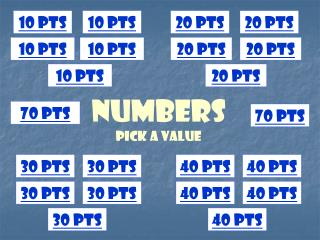DownloadDownload Presentationnumbers Pick a Value

# numbers Pick a Value

Download Presentation## numbers Pick a Value

- - - - - - - - - - - - - - - - - - - - - - - - - - - E N D - - - - - - - - - - - - - - - - - - - - - - - - - - -
##### Presentation Transcript

1. 10 pts 10 pts 20 pts 20 pts 10 pts 10 pts 20 pts 20 pts 10 pts 20 pts numbersPick a Value 70 pts 70 pts 30 pts 30 pts 40 pts 40 pts 30 pts 30 pts 40 pts 40 pts 30 pts 40 pts

2. What are the next three numbers?452, 453, 454, ___, ___, ___ A. 448, 449, 450 B. 452, 453, 454 C. 455, 456, 457 D. 455, 454, 453

3. What are the next three numbers?905, 906, 907, ___, ___, ___ A. 906, 907, 908 B. 908, 909, 910 C. 901, 902, 903 D. 908, 907, 906

4. What are the first three numbers?___, ___, ___, 564, 565, 566 A. 561, 562, 563 B. 563, 563, 563 C. 567, 568, 569 D. 561, 560, 559

5. What are the first three numbers? ___, ___, ___, 700, 701, 702 A. 703, 704, 705 B. 699, 700, 701 C. 697, 698, 699 D. 700, 701, 702

6. In the following number, which place is the 2 in? 921 A. thousands place B. hundreds place C. tens place D. ones place

7. In the following number, which place is the 7 in?758 A. thousands place B. hundreds place C. tens place D. ones place

8. Round to the nearest hundred: 458 A. 400 B. 500 C. 300 D. 600

9. Round to the nearest hundred: 812 A. 900 B. 600 C. 1,000 D. 800

10. Choose the correct symbol. ( <, = ,> )670 607 A. < B. > C. =

11. Choose the correct symbol. ( <, = ,> )212 212 A. < B. > C. =

12. Choose the correct symbol. ( <, = ,> )602 206 A. < B. > C. =

13. Choose the correct symbol. ( <, = ,> )909 999 A. < B. > C. =

14. Put these numbers in order from least to greatest.142 19 356 217 A. 356, 217, 142, 19 B. 19, 217, 142, 356 C. 19, 142, 217, 356

15. Put these numbers in order from least to greatest.205 4 714 38 A. 4, 38, 205, 714 B. 205, 4, 714, 38 C. 4, 205, 714, 38

16. Put these numbers in order from greatest to least.981 217 918 271 A. 217, 271, 918, 981 B. 981, 217, 271, 918 C. 981, 918, 271, 217

17. Put these numbers in order from least to greatest.121 12 201 102 A. 121, 12, 201, 102 B. 12, 102, 121, 201 C. 201, 121, 102, 12

18. Which shows the same value as 941? A. 900 + 40 B. 900 + 1 C. 900 + 40 + 1

19. Which shows the same value as 809? A. 800 + 90 B. 800 + 9 C. 800 + 900

20. Which shows the same value as: 600 + 50 + 6 A. 656 B. 606 C. 665

21. Which shows the same value as: 300 + 2 A. 203 B. 320 C. 302

22. Alice sold 120 lollipops for the school fundraiser. Jaime sold 274 lollipops. ABOUT how many lollipops did the two girls sell in all? A. 120 lollipops B. 200 lollipops C. 300 lollipops D. 400 lollipops

23. DJ Ray owns 543 CDs. He gave his brother 189 CDs to get started as a DJ. ABOUT how many CDs does DJ Ray have left? A. 543 CDs B. 300 CDs C. 700 CDs D. 400 CDs

24. Nice work! You’re an ACE!

25. SorryTry again!